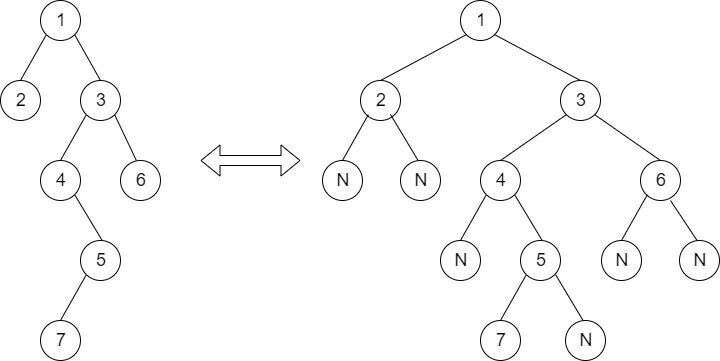Level order traversal in spiral form
##### Submissions: 38970   Accuracy: 43.27%   Difficulty: Easy   Marks: 2

Complete the function to print spiral order traversal of a tree. For below tree, function should print 1, 2, 3, 4, 5, 6, 7.Input Format:
First line of input contains the number of test cases T. For each test case, there will be only a single line of input which is a string representing the tree as described below:

1. The values in the string are in the order of level order traversal of the tree where, numbers denotes node values, and a character “N” denotes NULL child.

2. For example:For the above tree, the string will be: 1 2 3 N N 4 6 N 5 N N 7 N

Output Format:
The function should print level order traversal in spiral form.

The task is to complete the function printSpiral() which prints the elements in spiral form of level order traversal. The newline is automatically appended by the driver code.

Constraints:
1 <= T <= 30
1 <= Number of nodes <= 105
1 <= Data of a node <= 105

Example:
Input:

2
1 3 2
10 20 30 40 60
Output:

1 3 2
10 20 30 60 40

Explanation:
Testcase1: The tree is
1
/      \
3       2
So, the spiral level order would be 1 3 2
Testcase2: The tree is
10
/        \
20         30
/       \
40       60
So, the spiral level order would be 10 20 30 60 40

Note: The Input/Ouput format and Example given are used for system's internal purpose, and should be used by a user for Expected Output only. As it is a function problem, hence a user should not read any input from stdin/console. The task is to complete the function specified, and not to write the full code.

#### ** For More Input/Output Examples Use 'Expected Output' option **

Contributor: Amit Khandelwal, Harshit Sidhwa
Author: Shubham Joshi 1

If you have purchased any course from GeeksforGeeks then please ask your doubt on course discussion forum. You will get quick replies from GFG Moderators there.